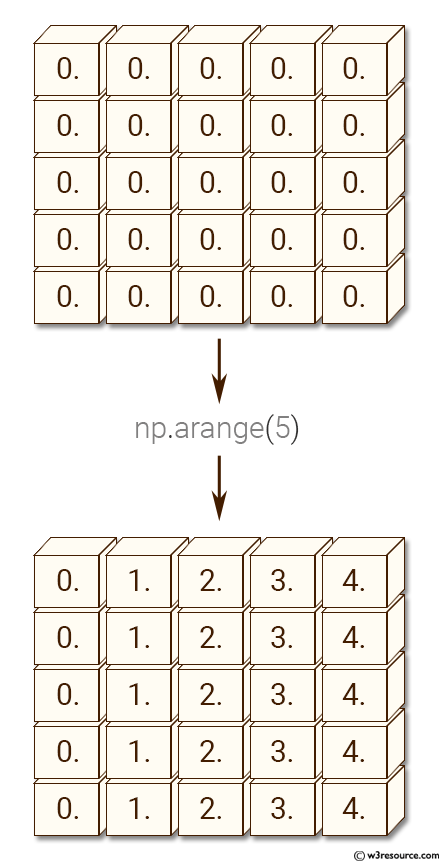﻿ NumPy: Create a 5x5 matrix with row values ranging from 0 to 4 - w3resource# NumPy: Create a 5x5 matrix with row values ranging from 0 to 4

## NumPy: Array Object Exercise-64 with Solution

Write a NumPy program to create a 5x5 matrix with row values ranging from 0 to 4.

Pictorial Presentation:Sample Solution:-

Python Code:

``````import numpy as np
x = np.zeros((5,5))
print("Original array:")
print(x)
print("Row values ranging from 0 to 4.")
x += np.arange(5)
print(x)
```
```

Sample Output:

```Original array:
[[ 0.  0.  0.  0.  0.]
[ 0.  0.  0.  0.  0.]
[ 0.  0.  0.  0.  0.]
[ 0.  0.  0.  0.  0.]
[ 0.  0.  0.  0.  0.]]
Row values ranging from 0 to 4.
[[ 0.  1.  2.  3.  4.]
[ 0.  1.  2.  3.  4.]
[ 0.  1.  2.  3.  4.]
[ 0.  1.  2.  3.  4.]
[ 0.  1.  2.  3.  4.]]
```

Python Code Editor:

Have another way to solve this solution? Contribute your code (and comments) through Disqus.

What is the difficulty level of this exercise?

Test your Python skills with w3resource's quiz

﻿

## Python: Tips of the Day

List comprehension:

```>>> m = [x ** 2 for x in range(5)]
>>> m
[0, 1, 4, 9, 16]
```